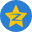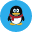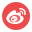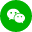X

# php 统计网页打开耗时和脚本运行内存

php计算页面`执行时间耗时`，计算方式是运行开始结束时间减去运行开始时间。

如，在php代码脚本的最顶部代码开始时，获取时间戳并保存到变量，再在代码的底部结束时获取一次时间戳，相减，即是整个脚本代码执行的时间。用到`microtime()`函数。

而获取当前php`脚本消耗的内存`大小的方式和获取时间的原理一样，用到的`memory_get_usage（）`函数。

```<?php

//开始时间计算
\$se = explode(' ',microtime()); //返回数组，当前时间微秒数和时间戳秒数
\$ntime = \$se + \$se;

/*
*中间的代码
*/

\$se1 = explode(' ',microtime());//代码结束计算当前秒数
\$etime = \$se1 + \$se1;

\$htime = \$etime - \$ntime; //代码执行结束时间 - 代码开始时间 = 执行时间
\$hstime = round(\$htime,3);//获取小数点后三位

?>```

```<?php
\$memory = 0;
//开始内存
\$memory_start = function_exists('memory_get_usage')?memory_get_usage():'0';

/*
*中间的代码
*/

//脚本执行完获取内存
\$memory_end = function_exists('memory_get_usage')?memory_get_usage():'0';

//脚本消耗内存
\$memory = \$memory_end - \$memory_start;

?>```表情：